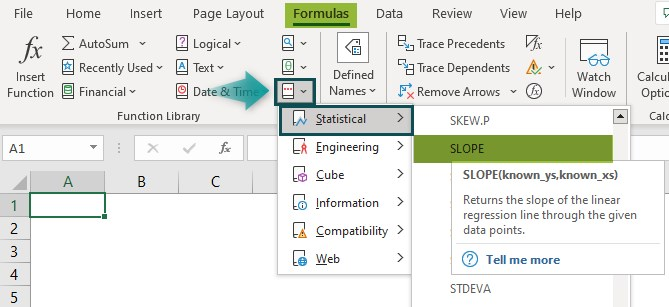# SLOPE Function In ExcelArticle byExcelMojo Team## What Is SLOPE Function In Excel?

The SLOPE function in Excel evaluates the slope of a line between 2 data points, generated by linear regression. The slope is the vertical or horizontal distance between any two points on this line. The function describes the correlation of two numeric variables and how any change in the independent variable affects the dependent variables.

The SLOPE function in Excel is an inbuilt Statistical function, which means we can insert it as a formula from the “Function Library” or enter it directly in the worksheet. For example, we will apply the SLOPE formula to calculate the slope using the x and y values. Select cell B4, enter the formula =SLOPE(B2:B3,A2:A3), and press the “Enter” key.

The output is “2”, as shown above.

###### Key Takeaways
• The SLOPE function in Excel calculates the slope of a line between the given data points, the known_xs and the known_ys values, which can be pictorially represented using a Line graph. The steeper the line, the larger the slope.
• The length of the known_xs value should be the same as the known_ys value, and the variance of the known_xs must not be zero.
• Cells with the value ‘0’ are considered, but the cells or cell range that are blank/empty, or cells with text and logical values are ignored.
• The arguments must be numbers, names, arrays, or references containing numbers.

### SLOPE() Excel Formula

The syntax of the SLOPE Excel formula is,

The arguments of the SLOPE Excel formula are,

• known_ys: It is a mandatory argument. It is an array or range of numerically dependent data points.
• known_xs: It is a mandatory argument. It is the set of independent data points.

### How To Use SLOPE Excel Function?

We can use the SLOPE function in Excel in 2 methods, namely,

1. Access from the Excel ribbon.
2. Enter in the worksheet manually.

#### #1 – Method – Access from the Excel ribbon

First, choose an empty cell – select the “Formulas” tab – go to the “Functions Library” group – click the “More Functions…” option drop-down – click the “Statistical” options right arrow – select the “SLOPE” function, as shown below.

The “Function Arguments” window appears. Enter the “known_ys & known_xs” as cell values, Excel cell references, or cell range in the argument fields à click “OK”, as shown below.

#### #2 – Method – Enter in the worksheet manually

1. Select an empty cell for the output.
2. Type =SLOPE( in the selected cell. [Alternatively, type “=S” and double-click the SLOPE function from the list of suggestions shown by Excel.
3. Enter the argument values and close the brackets.
4. Press the “Enter” key.

Let us take an example to understand this function. We will calculate the slope from the x & y values using SLOPE function in Excel.

In the table, the data is,

• Column A contains the known_xs.
• Column B contains the known_ys.

The steps to evaluate the slope values using SLOPE function in Excel are as follows:

1. Select cell B6 and enter the formula =SLOPE(B2:B5 i.e., the known y value.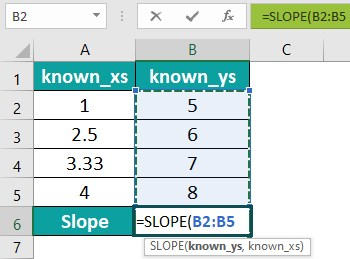2. Put a comma ‘,’, enter the value of ‘known_xs’ as “A2:A5”, and close the brackets. The complete formula is =SLOPE(B2:B5,A2:A5).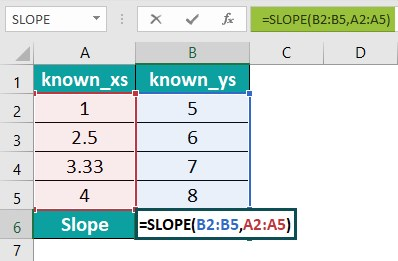3. Press the “Enter” key. The result is “0.979733”, as shown below.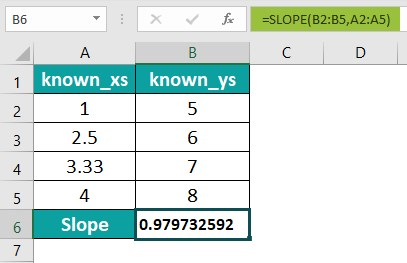4. To represent the output in a Line graph, choose cell range A2:B6 – select the “Insert” tab – go to the “Charts” group – click the “Insert Line or Area Chart” option drop-down – in the “2-D Line” group, and select the first “Line” chart, as shown below.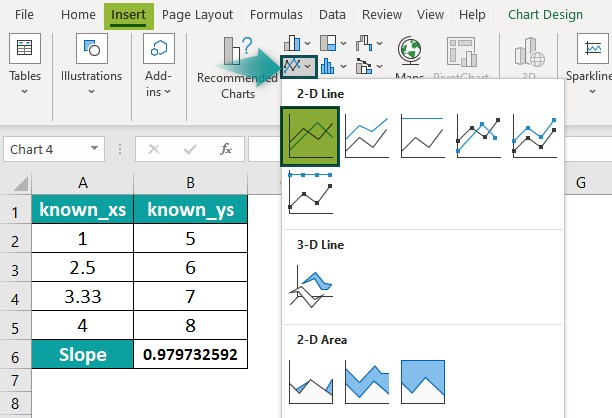The output is shown below with a visible SLOPE in the Line graph.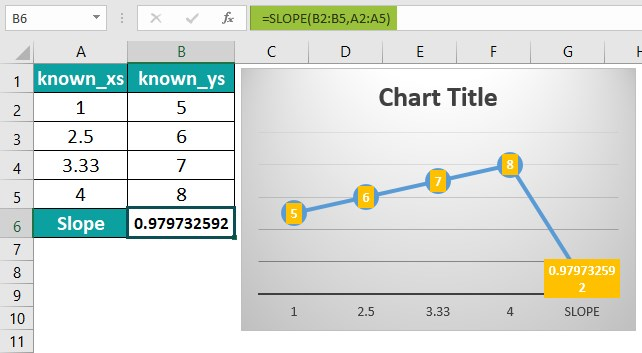### Examples

We can understand the SLOPE function in Excel examples with some advanced scenarios.

#### #1 – Example

We will calculate the slope from the values using the SLOPE function in Excel.

In the table, the data is,

• Column A contains the known_xs.
• Column B contains the known_ys.

The steps to evaluate the slope values by the SLOPE function are as follows:

1: Select cell B11, enter the formula =SLOPE(B2:B10,A2:A10) [“B2:B10”, i.e., the known y value, and “A2:A10”, i.e., the known x value.], and press the “Enter” key.

The result is “0.137885512”, as shown in the above image.

2: Let us create a Line graph. First, choose cell range A2:B11 – select the “Insert” tab – go to the “Charts” group – click the “Insert Line or Area Chart” option drop-down – in the “2-D Line” group, and select the first “Line” chart. We will get the following Line chart automatically.

#### #2 – Example

We will calculate the slope from the values using the SLOPE function.

In the table, the data is,

• Column A contains Months.
• Column B contains the known_xs.
• Column C contains the known_ys.

The steps to evaluate the slope values by the SLOPE function in Excel are as follows:

1: Select cell C14, enter the formula =SLOPE(C2:C13,B2:B13), and press the “Enter” key.

The result is “0.525436”, as shown in the above image.

2: Let us create a Line graph. First, choose cell range A2:C14 – select the “Insert” tab – go to the “Charts” group – click the “Insert Line or Area Chart” option drop-down – in the “2-D Line” group, and select the first “Line” chart. We will get the following Line chart automatically.

#### #3 – Example

We will calculate the slope using the SLOPE function in Excel for the employee salaries.

In the table, the data is,

• Column A contains the known_xs.
• Column B contains the known_ys.

The steps to evaluate the slope values by the SLOPE function in Excel are as follows:

1: Select cell B11, enter the formula =SLOPE(B2:B10,A2:A10), and press the “Enter” key.

The result is “4084989.962”, as shown in the above image.

2: Let us create a Line graph. First, choose cell range A2:B11 – select the “Insert” tab – go to the “Charts” group – click the “Insert Line or Area Chart” option drop-down – in the “3-D Line” group, and select the “Line” chart. We will get the following Line chart automatically.

### Important Things To Note

• We get the “#N/A!” error, when the given array of known_xs array of known_ys are of different lengths.
• The “#DIV/0!” error occurs,
• When the variance of the given known_xs evaluates to zero.
• When any of the given arrays known_xs or known_ys values are empty.
• When we have only one row of argument values.
• The “#VALUE!” error occurs when one of the values, i.e., x or y, is empty or blank.

1. What does the SLOPE function do in Excel?

The SLOPE function in Excel is a statistical function that returns the slope of a straight line between given data points in known_ys and known_xs values. The slope of a linear regression line is the vertical or horizontal distance between any of the two points on this line.

The syntax of the SLOPE function is =SLOPE(known_ys,known_xs)

2. How does the SLOPE function work in Excel?

We can work with the SLOPE function in Excel as follows:
1. Select an empty cell for the output.
2. Type =SLOPE( in the selected cell. [Alternatively, type “=S” and double-click the 3. SLOPE function from the list of suggestions shown by Excel.]
4. Enter the argument values and close the brackets.
5. Press the “Enter” key.

For example, we will calculate the slope from the x and y values using the SLOPE function in Excel.

The steps to evaluate the slope values by the SLOPE function are as follows:

Step 1: Select cell B6.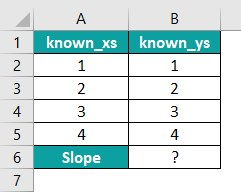Step 2: Enter the value of ‘known_ys’ as “B2:B5”, and the value of ‘known_xs’ as “A2:A5”. The complete formula is =SLOPE(B2:B5,A2:A5), and press the “Enter” key.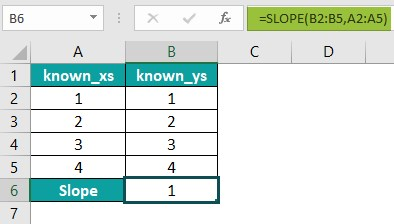The result is “1”, as shown above.

3. Where is the SLOPE function in Excel?

The SLOPE function in Excel in the “Formulas” tab, as is inserted as follows:

First, choose an empty cell – select the “Formulas” tab – go to the “Functions Library” group – click the “More Functions…” option drop-down – click the “Statistical” options right arrow – select the “SLOPE” function, as shown below.